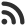Feed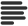Articles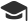Tutorials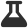CodingLab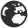CommunityLeaderboardFounder & Programmer at HackersFriend Updated Dec. 2, 2019, 12:46 a.m. ⋅ 81 views

# C++ Hacks for Competitive programming

There are lot of C++ Hacks you can use to save time in competitive programming. Here are few of them.

• Getting rid of “includes”: Just use
`#include <bits/stdc++.h> `
• Useful inbuilt functions:
• `__gcd(a, b):` Returns the GCD of a and b
• `__builtin_popcount(x)` : Returns the number of set bits in x
• Initializer Lists: These make STL containers easy to initialize, for eg:
• `vector<int> odd_nos = {1, 3, 5, 7, 9}; `
• `pair<int, string> p = {1, “Hi”}; //Equiv. to p=make_pair(1, "Hi")`
• `map<int, string> m = { {1, “This”}, {2, “is”}, {3, “awesome”} }; `
• Finding min and max of multiple variables:
``````//Long Way:

int max_of_3 = max(a, max(b, c));
int max_of_4 = max(max(a, b), max(c, d));

//Easier Way - Can be extended to any number of variables:

int max_of_3 = max({a, b, c});
int max_of_4 = max({a, b, c, d});``````

• Range-based for loops: Makes it much simpler to iterate through containers.
``````//Old Way:
for(auto it=container.begin(), it!=container.end(); it++)
cout<<*it<<" ";

//Alternatively:
for(int i=0;i<container.size();i++) //If the container is a vector
cout<<container[i]<<" ";

//Easier Way:
for(auto &it:container) //Using & also allows us to modify the elements
cout<<it<<" ";``````

• Tie and Swap:
• Tie makes it easier to initialize multiple variables in a single line
• Swap enables swapping of variables, and even containers with a single statement
``````//Initializes a with -1, b with 1, etc
tie(a, b, c, d) = make_tuple(-1, 1, -2, 2);

//x, y can be two integers, or two vectors, or any two containers
swap(x, y);
``````
• Macros:
• If you are tired of typing some statement like `push_back` again and again, you can just use `#define pb push_back` , and type `pb` in your code.
• Getting the name of the argument in macro using #, and using it to debug your program:
``````#define trace(x) cerr<<#x<<": "<<x<<" "<<endl;

int main()
{
int a=5;
trace(a); //prints: "a: 5" to stderr stream
}``````
• C++ allows templates with variable number of arguments. If you are tired of writing trace1, trace2, trace3, etc for different number of variables you want to print in a line, then you can read about Variadic Functions Templates here.
• Code for debugging variable number of parameters: Here.

 arrow_upward UpvoteComment arrow_downward downvote

Events

Nov. 28, 2018, 5:30 p.m.

Python from zero to hero

place Delhi

Aug. 13, 2018, 5:30 p.m.

Python from zero to hero

place Bangalore ( HackersFriend office BTM Layout)